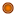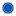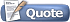Thread: The Project Euler thread View Single Post10-21-2011, 12:00 PM #13 iironiic Diana is my queen <3Join Date: Jan 2009 Age: 28 Posts: 4,183Re: THE project euler thread @leonid: I got an answer to #80 but I don't understand why it's not the correct answer. Can you help me figure out the problem in my code? Just to see if there is something wrong in my code, I tried using my program to help me determine some values. Let's define the digital sum of the first 100 digits of radical n to be f(n). Are these values correct? I chose a few test numbers just to see if my program is telling me the right digital sum. It did tell me that f(2) = 475 which was given in the problem. f(3) = 441 f(7) = 398 f(35) = 440 f(69) = 439 f(95) = 460 Any help would be appreciated. Thanks! __________________